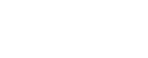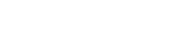## 什幺是tensorflow.js

.js是一个能运行在浏览器和nodejs的一个机器学习、机器训练的javascript库，众所周知在浏览器上用javascript进行计算是很慢的，而tensorflow.js会基于WebGL通过gpu进行计算加速来对高性能的机器学习模块进行加速运算，从而能让我们前端开发人员能在浏览器中进行机器学习和训练神经网络。本文要讲解的项目代码，就是要根据一些规则模拟数据，然后通过机器学习和训练，根据这些数据去反向推测出生成这些数据的公式函数。

## 基本概念

### 张量（tensors）

```const tensors = tf.tensor([[1.0, 2.0, 3.0], [10.0, 20.0, 30.0]]);
tensors.print();```tensorflow还提供了语义化的张量创建函数：tf.scalar（创建零维度的张量）, tf.tensor1d（创建一维度的张量）, tf.tensor2d（创建二维度的张量）, tf.tensor3d（创建三维度的张量）、tf.tensor4d（创建四维度的张量）以及 tf.ones（张量里的值全是1）或者tf.zeros（张量里的值全是0）。

tensorflow还提供了语义化的张量创建函数：tf.scalar（创建零维度的张量）, tf.tensor1d（创建一维度的张量）, tf.tensor2d（创建二维度的张量）, tf.tensor3d（创建三维度的张量）、tf.tensor4d（创建四维度的张量）以及 tf.ones（张量里的值全是1）或者tf.zeros（张量里的值全是0）。

### 变量（variable）

```const initialValues = tf.zeros();//[0, 0, 0, 0, 0]
const biases = tf.variable(initialValues); //通过张量初始化变量
biases.print(); //输出[0, 0, 0, 0, 0]```

### 操作（operations）

```const e = tf.tensor2d([[1.0, 2.0], [3.0, 4.0]]);
const f = tf.tensor2d([[5.0, 6.0], [7.0, 8.0]]);
e_plus_f.print();```### 内存管理（dispose和tf.tiny）

dispose和tf.tiny都是用来清空GPU缓存的，就相当于咱们在编写js代码的时候，通过给这个变量赋值null来清空缓存的意思。

```var a = {num: 1};
a = null;//清除缓存```

### dispose

```const x = tf.tensor2d([[0.0, 2.0], [4.0, 6.0]]);
const x_squared = x.square();
x.dispose();
x_squared.dispose();```

### tf.tiny

```const average = tf.tidy(() => {
const y = tf.tensor1d([4.0, 3.0, 2.0, 1.0]);
const z = tf.ones();
return y.sub(z);
});
average.print()```## 模拟数据## 设置变量（Set up variables）

```const a = tf.variable(tf.scalar(Math.random()));
const b = tf.variable(tf.scalar(Math.random()));
const c = tf.variable(tf.scalar(Math.random()));
const d = tf.variable(tf.scalar(Math.random()));```

```let a = Math.random();
let b = Math.random();
let c = Math.random();
let d = Math.random();```## 创建优化器（Create an optimizer）

```const learningRate = 0.5;
const optimizer = tf.train.sgd(learningRate);```

learningRate这个变量是定义学习率，在进行每一次机器训练的时候，会根据学习率的大小去进行计算的偏移量调整幅度，学习率越低，最后预测到的值就会越精准，但是响应的会增加程序的运行时间和计算量。高学习率会加快学习过程，但是由于偏移量幅度太大，容易造成在正确值的周边上下摆动导致运算出的结果没有那幺准确。

tf.train.sgd是我们选用了tensorflow.js里帮我们封装好的SGD优化器，即随机梯度下降法。在机器学习算法的时候，通常采用梯度下降法来对我们的算法进行机器训练，梯度下降法常用有三种形式BGD、SGD以及MBGD。SGD它是利用每个样本的损失函数对θ求偏导得到对应的梯度，来更新θ：## 预期函数模型（training process functions）

```function predict(x) {
// y = a * x ^ 3 + b * x ^ 2 + c * x + d
return tf.tidy(() => {
return a.mul(x.pow(tf.scalar(3, 'int32')))
});
}```

a.mul(x.pow(tf.scalar(3, ‘int32’)))就是描述了a*x^3（a乘以x的三次方），b.mul(x.square()))描述了b * x ^ 2（b乘以x的平方），c.mul(x)这些同理。注意，在predict函数return的时候，用tf.tidy包了起来，这是为了方便内存管理和优化机器训练过程的内存。

## 定义损失函数（loss）```function loss(prediction, labels) {
const error = prediction.sub(labels).square().mean();
return error;
}```

## 机器训练（training）

```const numIterations = 75;
async function train(xs, ys, numIterations) {
for (let iter = 0; iter < numIterations; iter++) {
//优化器：SGD随机梯度下降
optimizer.minimize(() => {
const pred = predict(xs);
//损失函数：MSE均方误差
return loss(pred, ys);
});
//防止阻塞浏览器
await tf.nextFrame();
}
}```

```import {generateData} from './data';//这个文件在git仓库里
const trainingData = generateData(100, {a: -.8, b: -.2, c: .9, d: .5});
await train(trainingData.xs, trainingData.ys, 75);```

```console.log('a', a.dataSync());
console.log('b', b.dataSync());
console.log('c', c.dataSync());
console.log('d', d.dataSync());```

## 项目运行和安装

```git clone https://github.com/tensorflow/tfjs-examples
cd tfjs-examples/polynomial-regression-core
yarn
yarn watch```

## 结语

bb了这幺多，本来不想写结语的，但是想想，还是想表达一下本人内心的一个搞笑荒谬的想法。我为什幺会对这个人工智能的例子感兴趣呢，是因为，在我广西老家（一个偏远的山村），那边比较封建迷信，经常拿一个人的生辰八字就去计算并说出这个人一生的命运，balabala说一堆，本人对这些风气一贯都是嗤之以鼻。但是，但是，但是。。。。荒谬的东西来了，我老丈人十早年前因为车祸而断了一条腿，几年前带媳妇和老丈人回老家见亲戚，老丈人觉得南方人这些封建迷信很好玩，就拿他自己的生辰八字去给乡下的老者算了一下，结果那个老人说了很多，并说出了我老丈人出车祸的那一天的准确的日期，精确到那一天的下午大致时间。。。。。这。。。。这就好玩了。。。当年整个空气突然安静的场景至今历历在目，这件事一直记在心里，毕竟我从来不相信这些鬼鬼乖乖的东西，一直信奉科学是至高无上带我们飞的唯一真理，但是。。。真的因为这件事，让我菊紧蛋疼不知道怎幺去评价。。。。